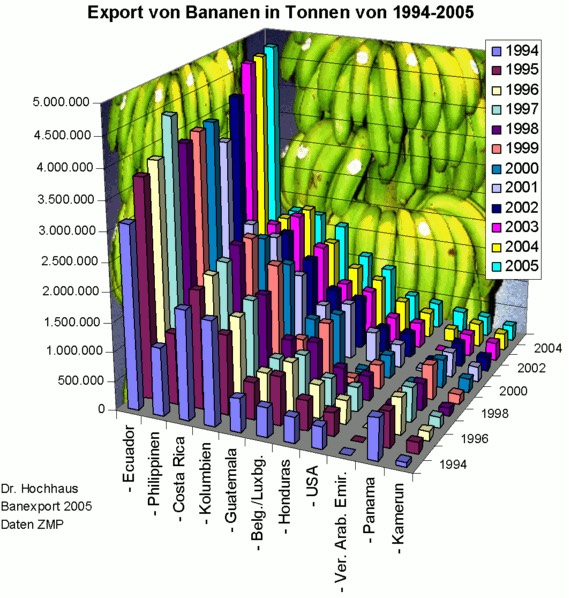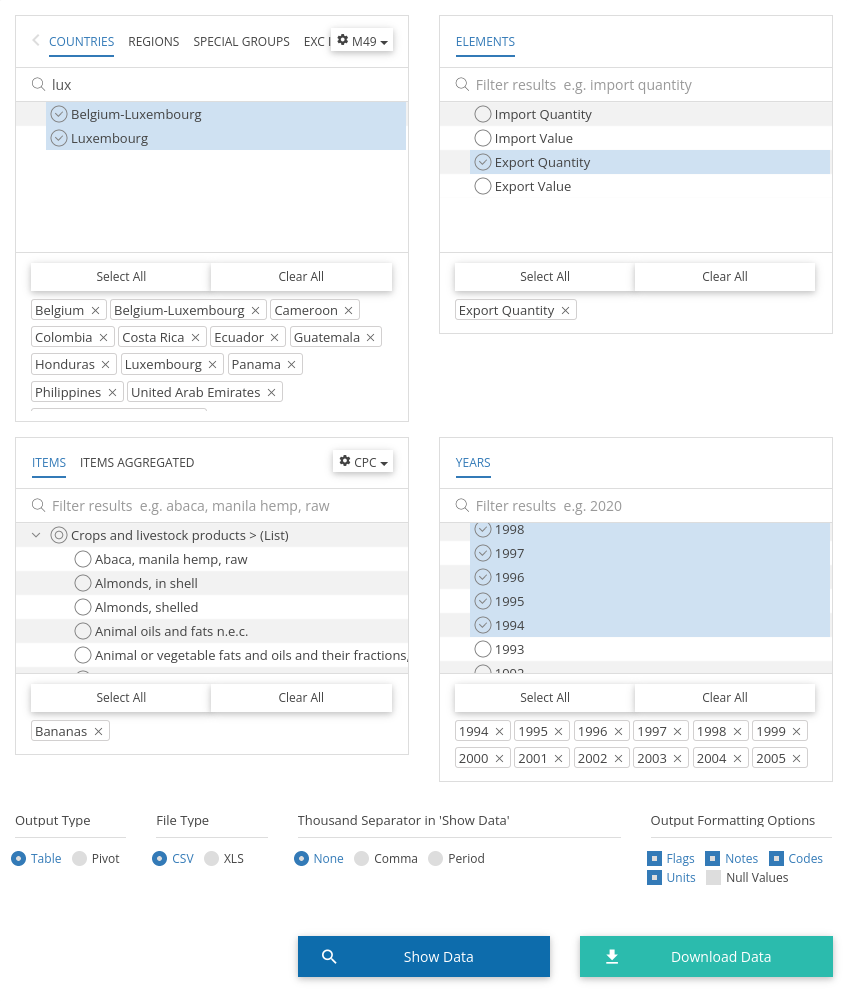# Export in Bananen in Tonnen von 1994-2005 (Banana exports in tonnes from 1994-2005)

R
Ggplot2
Ggplot2 effective visualization.
Author

Mauricio “Pachá” Vargas S.

Published

December 21, 2022

Updated 2022-05-28: I moved the blog to Quarto, so I had to update the paths.

A friend who doesn’t use the Tidyverse sent me this very nice plot:My first intuition to obtain the data for this unidentified plot was to go to FAO, and it was there!

I went to FAO Stat, filtered the countries and years seen in the plot and I got the required inputs to re-express the information.Now it’s time to use the Tidyverse, or at least parts of it. The resulting datasets from the in-browser filters is here.

``````library(ggplot2)
library(dplyr)
library(forcats)

message(getwd())

mutate(
Year2 = fct_relevel(
substr(Year, 3, 4), c(94:99, paste0("0", 0:5))),
Area = case_when(
Area %in% c("Belgium","Luxembourg") ~ "Belgium-Luxembourg",
TRUE ~ Area
)
) %>%
group_by(Year2, Area) %>%
summarise(Value = sum(Value, na.rm = T))

ggplot(bananas) +
geom_col(aes(x = Year2, y = Value), fill = "#f5e41a") +
facet_wrap(~ Area, ncol = 3) +
labs(
x = "Year",
y = "Value (tonnes)",
title = "Export in Bananen in Tonnen von 1994-2005\n(Banana exports in tonnes from 1994-2005)",
subtitle = "Source: Unidentified"
) +
theme_minimal(base_size = 13) +
scale_y_continuous(labels = scales::label_number(suffix = " M", scale = 1e-6))``````

The challenges were:

• Combine Belgium and Luxembourg data into a single area
• Express the axis in millions of tonnes
• Find a right banana yellow for the plot

I hope it’s less cluttered than the original plot!